# What is Kurtosis?

Kurtosis is a parameter of any signal, which represents its “peakedness”. But why is this parameter important for vibration testing and data analysis? And how can you manipulate it in RULA software? Let’s find out.

In simple words, kurtosis is a measure of how steep or flat the peak of the curve is. If kurtosis is high, there are more peaks in the signal and their amplitudes are greater. In the most commonly used Random signal with Gaussian distribution the value of kurtosis is three.

Mathematically, the value of kurtosis is calculated by the formula: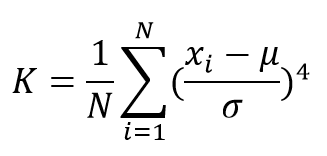Figure 1: Formula of kurtosis

where:

• μ - an average of the signal
• σ - standard deviation of the signal
• x - signal values

The figures below show the time waveforms of acceleration signals with Kurtosis values of three and eight.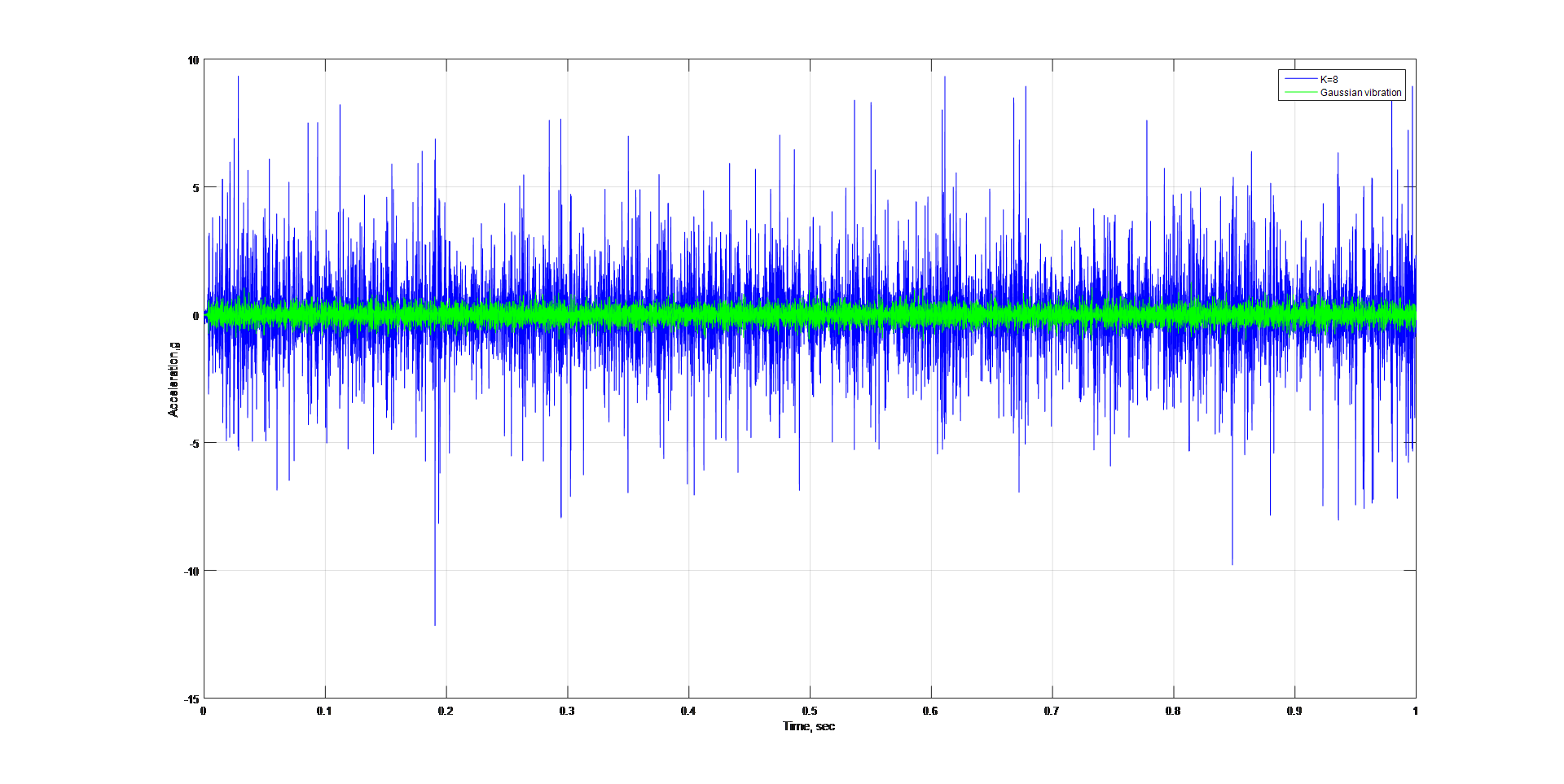Figure 2: Waveforms of acceleration with different Kurtosis value in RULA software

There are two probability density lines plotted on the figure below. The blue one is for the signal with the kurtosis value of 8, and the green one – for the value of 3.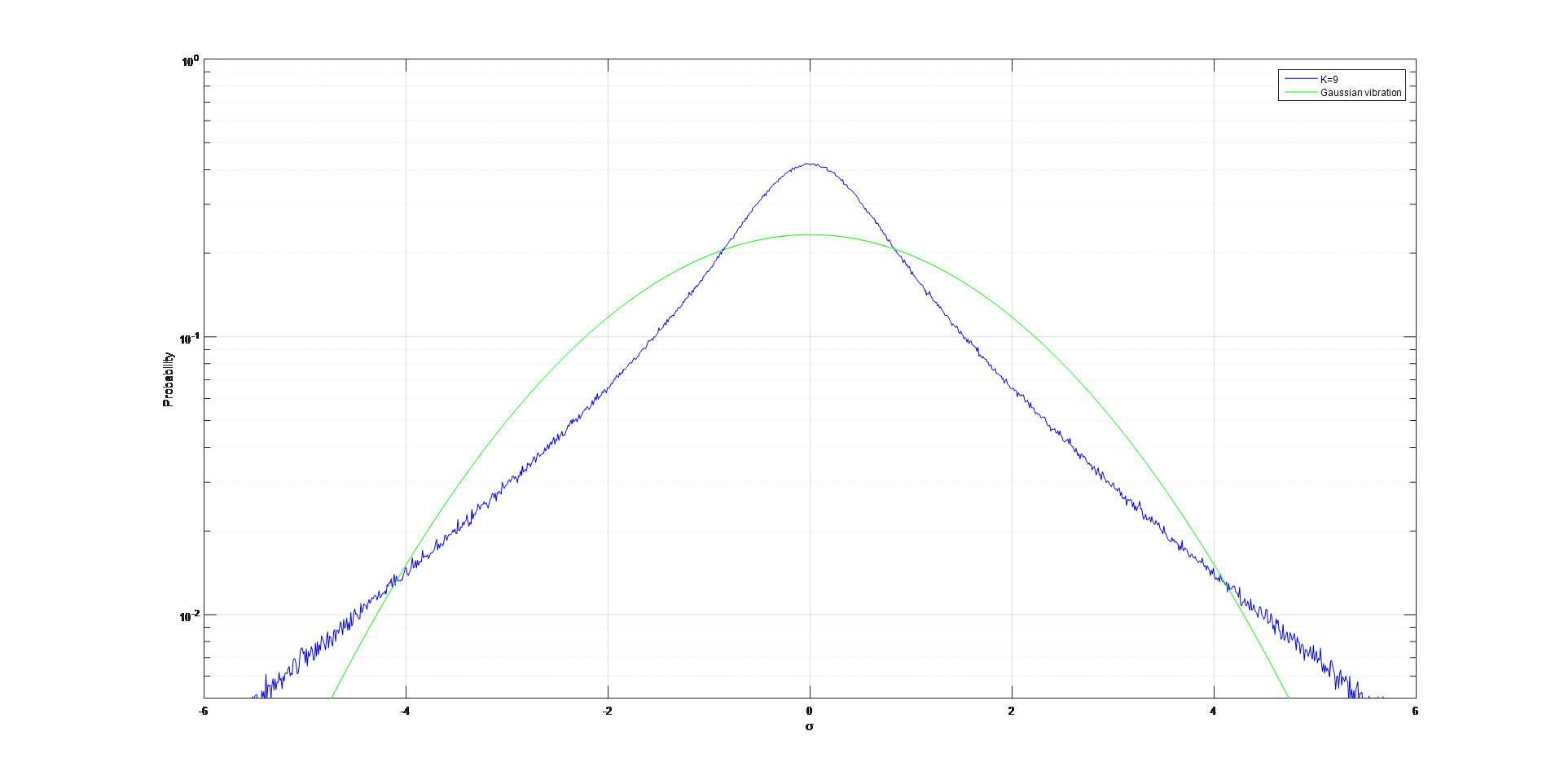Figure 3: Plot of density lines with different kurtosis values in RULA software

### Why is Kurtosis Important?

In real-life conditions, a lot of vibrations are characterized by signals with a kurtosis value higher than three (Gaussian random). The fatigue damage potential of such vibration is greater than that of a traditional Gaussian Random signal. Therefore, using a pure Gaussian Random will actually undertest the structure. Adjusting the value of Kurtosis offers an easy solution to this problem.

### Kurtosis settings in VisProbe SL

To enable kurtosis control, navigate to the Control tab of the Test Settings window.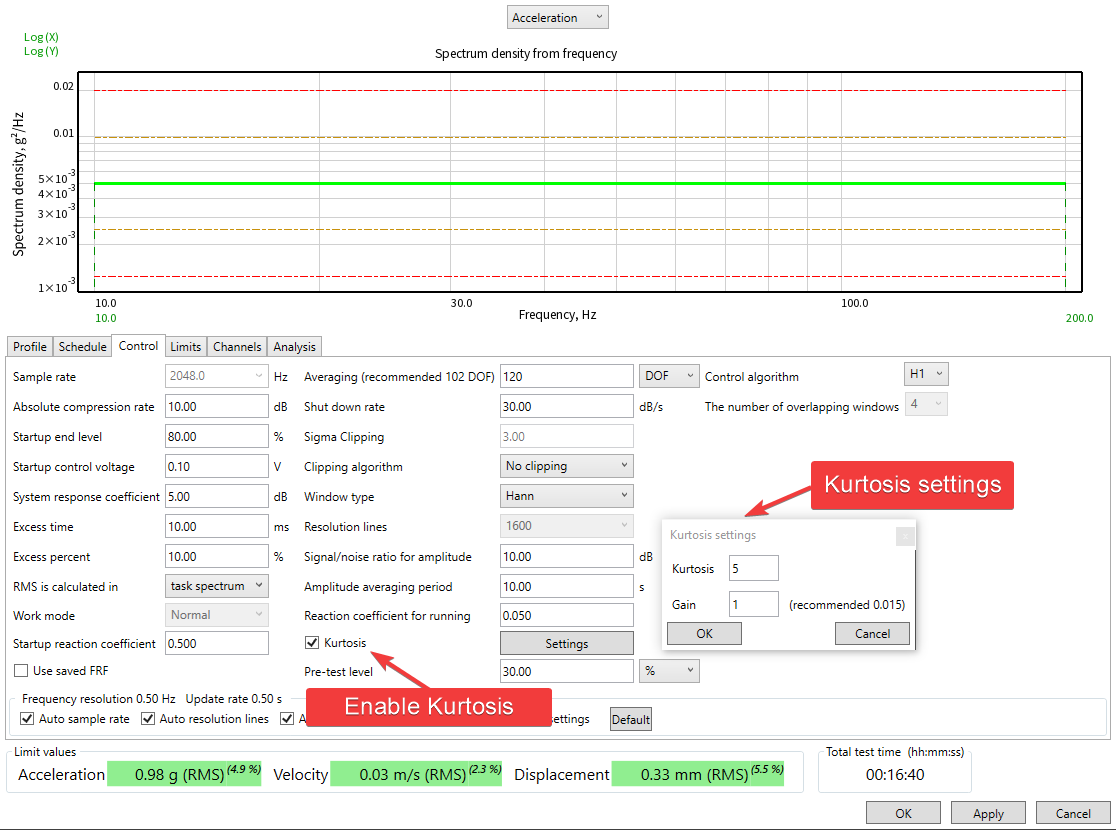Figure 4: Enabling Kurtosis control in RULA sofware

In the tab, check the Kurtosis box. Then press the Settings button and specify the value of the Kurtosis and Gain parameters.

Fill the Kurtosis field with the desired value of kurtosis. The Gain parameter allows you to adjust the speed of feedback reaction of the Kurtosis control loop.

After the parameters are specified, RULA software may ask you to increase the sigma-clipping limit to make sure that the desired kurtosis value is feasible.

If increasing the sigma clipping limit does not help, the user can disable the clipping.

To see the kurtosis value during the test, create a Kurtosis plot: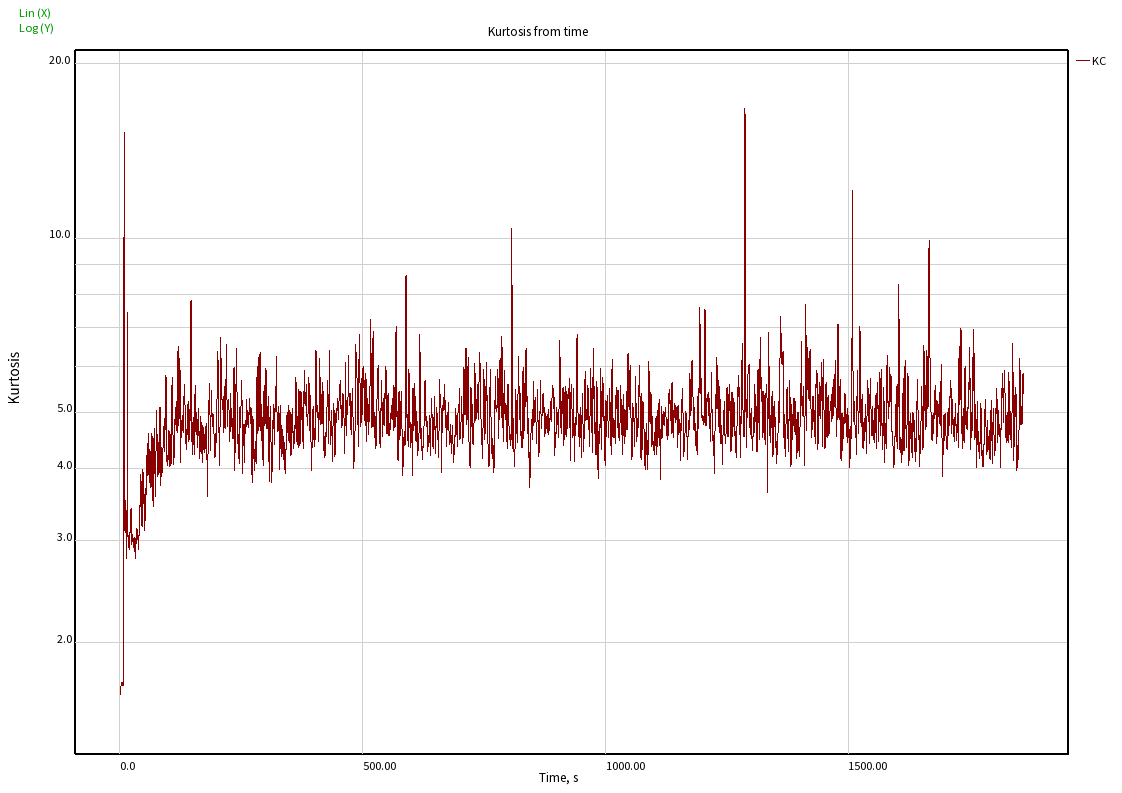Figure 5: Kurtosis plot in RULA software